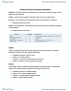STAT151 Chapter Notes - Chapter 15: Confidence Interval, Point Estimation, Standard Deviation

73 views4 pages
School
Department
Course
Professorsalmonmarten267 and 7 others unlocked4
Verified Note
4 documents

Document Summary

Make inferences about the whole population from sample data. Use one characteristic from the sample to represent the population. Sample proportion is a point estimation of p, the population probability for success. Head sample mean, point estimate for population mean u. Gives a single value that is supposed to be close to it"s true value. Tell us how close the estimate is. Different likely values of a point estimate from sample to sample , building an. Interval estimation upper and a lower bound for confidence intervals. When we don"t know p or sigma, we can use sample statistics to estimate these population parameters, and whenever the standard deviation is estimated, it is known as a standard error. Estimating the standard deviation of a sampling distribution. Entire intervals of reasonable values used , taking error and uncertainty into. The standard error for a sample proportion. The standard error for a sample mean.